## Abstracts

Here you can find some slides and the posters of the Workshop !!

## Minicourses:

Abstract: Linear Boltzmann equations describe a wide class of transport phenomena, in which  a population of independent particles or quasi-particles interacts with a background medium. In particular,  the homogeneous version corresponds to the Fokker-Planck equation of continuous time  Markov chains. Recently there have been a few attempts to formulate them as gradient flows, essentially in terms of so called energy variational inequalities. I will introduce a gradient flow approach based on an entropy dissipation inequality that can be applied naturally to the inhomogeneous case, and I will show  how the functional giving rise to the entropy dissipation inequality is related to the large deviation asymptotics of Markov chains.

Sabine Jansen, Gibbs point processes and cluster expansions

Abstract: Probability measures for configurations of points in R^d ("point processes") play an important role in both equilibrium statistical mechanics - as grand-canonical Gibbs measures - and spatial statistics. They also appear as stationary measures of random dynamics, for example, Markovian birth and death processes. The first part of the minicourse provides a short introduction to Gibbs point processes
geared towards briding the language gap between probability and analysis. The second part presents some recent developments on cluster expansions, i.e. in this context, Taylor series around the ideal gas (Poisson point process).

Patrícia Gonçalves, From microscopic to macroscopic: deriving deterministic laws from the random motion of particles

Abstract: In this mini-course I will present a method that allows to derive rigorously the macroscopic laws that rule the space-time evolution of the conserved quantities of a particle system. The goal is to describe the connection between the macroscopic equations and the microscopic system of particles. The former can be either PDEs or stochastic PDEs depending on whether one is looking at the law of large numbers or the central limit theorem; while the later is a collection of particles that move according to some random dynamics. Depending on the choice of the transition probability that particles obey, we will see that the macroscopic laws can be of different nature. I will present a model for which we can obtain the heat equation or the fractional heat equation depending on the type of transition probability that particles follow. Moreover, I will explain how to connect the particle system with different stochastic reservoirs  which will give rise, macroscopically, to different types of boundary conditions at the level of the PDEs.

## Special Lecture:

Barbara Rüdiger, Ludwig Eduard Boltzmann - The man who trusted atoms. Honoring the monograph of Carlo Cercignani

This talk is an attempt to honor the monograph of Carlo Cercignani (1939 - 2010) his successful engagement (despite his intensive well known research activity) in making more popular the physicist, mathematician and philosopher Ludwig Eduard Boltzmann (1844 - 1906). Based on the Monograph of Carlo Cercignani [C], in the first part of this talk I will describe the dispute of some scientist of the 19th century attacking the Boltzmann theory and his vision that thermodynamics has to be understood by connecting classical mechanics with the chaos theory. This monograph and other events motivated me to work recently on open problems related to the Boltzmann equation. In the second part of the talk I will describe some new result (in collaboration with S. Albeverio and P. Sundar [ARS], [ARSprep], as well as M. Friesen and P. Sundar [FRS]) related to the Boltzmann equation and the Enskog (- Boltzmann) equation, in particular in finding the stochastic processes associated.

References:

[C] Cercignani, C., Ludwig Boltzmann. The man who trusted atoms. With a foreword by Roger
Penrose. Oxford University Press, Oxford, 1998. xviii+329 pp

[ARS] Albeverio, S.; Rüdiger, B.; Sundar, P., The Enskog process. J. Stat. Phys. 167 (2017), no. 1, 90--122

[ARSprep] Albeverio, S.; Rüdiger, B.; Sundar, P. , The Boltzmann process, in preparation.

[FRS] Friesen, M., Rüdiger, B.; Sundar, P., The Enskog process for soft and hard potential, submitted to international journal, arXiv:1805.10141

## Invited talks:

Oriane Blondel, Convergence of a degenerate microscopic dynamics to the porous medium equation

Abstract: We consider an interacting particle system with nearest neighbor exchanges with a kinetic constraint. Gonçalves, Landim and Toninelli proved that, for initial densities uniformly bounded away from 0 and 1, the hydrodynamic limit is governed by the porous medium equation (PME).  In the present work, we consider the more general case where the density can take those
extreme values.  In this context, the PME solutions display a richer behavior, like moving interfaces, finite speed of propagation and breaking of regularity.   We therefore introduce a way to generalize the relative entropy method, using approximations of solutions to the hydrodynamic equation. This is a joint work with Clément Cancès, Makiko Sasada and Marielle Simon.

Gioia Carinci, Particle Systems and Free Boundary Problems

Abstract: There are several systems of interest in physics and biology which are related to free boundary problems in PDE's. In particular I will refer to the Brunet-Derrida models for biological selection mechanisms and the Fourier law for particles systems in domains which change in time due to reservoirs which fix the current (while usually it is the spatial domain and the density at the boundaries which are fixed). In both cases the hydrodynamic (or continuum) limit is described by parabolic equations with free boundaries of Stefan-type. I will give in this talk a short survey of the methods used to study such particle systems and the free boundary problems for the corresponding PDE's. Well known theorems on the Stefan problem yield a local existence for ''classical initial data''. I will introduce a new notion of relaxed solution for which global existence and uniqueness is proven for general initial data.

Diana Conache, Schonmann's projection from the one-sided point of view

Abstract: The projection of the two-dimensional Ising model onto a line, also known as the Schonmann projection, appeared as a physically natural example of a measure that was not Gibbs. In the framework of Dobrushin's program of restoration of Gibbsianness it was shown that this measure was weakly Gibbs, but it is still an open question if this is an almost Gibbs measure, i.e. if it is consistent with an almost continuous specification. We analyze this model from the one-sided conditioning point of view and prove that the Schonmann projection is an almost g-measure. We further establish some mixing properties, which ca be interpreted as a lack of one-sided wetting transition. Moreover, we discuss further open questions. This is joint work with S.A. Bethuelsen.

Xian Liao, Conserved energies for the one-dimensional Gross-Pitaevskii equation

Abstract: The one-dimensional Gross-Pitaevskii equation in this talk reads as one-dimensional defocusing cubic nonlinear Schroedinger equation,
but with nonzero boundary condition at infinity. I will first introduce
the solution space and endow it with a metric to make it a complete metric
space. Then I will establish a family of conserved energies which describe
the Sobolev regularities of the solution. The proof is based on the fact
that the 1d GP is completely integrable by means of inverse scattering
method and the associated transmission coefficient is invariant. We
analyze this invariant transmission coefficient to get an idea how to
establish those   energies. This is a joint work with Herbert Koch in
Bonn.

Constanza Rojas-Molina, Random Schrödinger operators in aperiodic media

Abstract: In this talk we will first give an introduction to the field of random Schrödinger operators, a standard framework for the study of disordered quantum systems. This theory was originally developed to explain rigorously the absence of electron propagation in random media, a phenomenon known as Anderson localization. We will then review some recent results on how this theory can be applied to study the propagation of electrons in aperiodic media, as for example, in quasicrystals.

Chiara Saffirio, From the many-body quantum dynamics to the Vlasov equation

Abstract: We review some results on the derivation of the Vlasov equation starting from a system of N interacting fermions, whose evolution is given by the N-particle Schrödinger equation. In order to get explicit bounds on the rate of convergence, we use the Hartree-Fock equation as a bridge between the Hamiltonian and the Vlasov dynamics. The results we present hold for smooth and singular interaction potentials.

Ariane Trescases, Regularity of the Boltzmann equation in bounded domains

Abstract: The Boltzmann equation (1872) models the evolution of a rarefied gas away from equilibrium. Many works treat the question of strong solutions close to equilibrium. However, only very few of them consider the case of a bounded domain, while this situation is the most natural in the applications. One major difficulty of this problem is the arising of a singularity on the trajectories grazing the boundary. We present here a study of the regularity of the Boltzmann equation in bounded domains. Thanks to the introduction of a kinetic distance which compensates the singularity at the boundary, we show the propagation of Sobolev norms and the C^1 propagation in convex domains. In non-convex domains, we show the propagation of BV regularity.

## Short invited talks:

Costanza Benassi, Loop Correlations in Random Wire Models

Abstract: Random loop models appear in a great variety of situations in both the probability and mathematical physics literature. For a wide class of them, a striking conjecture has been proposed: in dimensions three and higher, there should be phases with macroscopic loops and the joint distribution of their lengths is expected to be a member of the Poisson-Dirichlet distribution family. We propose a loop model which is strictly related to O(N) classical spin systems and which is an extension of the random current representation of the Ising model. We prove that even loop correlations are given by Poisson-Dirichlet.
Based on a joint work with D. Ueltschi, arXiv:1807.06564.

Mareike Lager, Random Matrices and Supersymmetry

Abstract: We consider random matrix models on a d-dimensional lattice Λ⊆Ζ^d.
Precisely, we study properties of the averaged density of states in the thermodynamic limit. A main role in the proofs plays the tool of supersymmetry. This is a joint work with M. Disertori.

Ilaria Maccari, Vortex physics in 2D disordered superconductors

Abstract: The study of quasi-two-dimensional (2D) superconductors can hardly avoid to take into account the presence of vortices. Many real systems, of both conventional and unconventional superconductors, undergoes the superconductor-insulator transition via the Brezinskii-Kosterlitz-Thouless (BKT) transition, at which the phase coherence of the condensate is destroyed by means of free-vortices proliferation. Quite interesting, the interplay between the vortex-unbinding and the electron inhomogeneity, which spontaneously emerges in thin SC films, makes the role of vortices even more crucial for a deeper understanding of the BKT signature. Indeed, in we have shown, by means of Monte Carlo simulations, that the disorder-induced granularity of the SC state, being spatially correlated, modifies the nucleation mechanism for vortexantivortex pairs leading to a considerable smearing of the universal superfluid-density jump, as compared to the paradigmatic clean case, in agreement with experimental observations. An other interesting issue concerns the fate of the SC transition in the presence of a finite flux of magnetic field. Indeed, in this case the transition is no longer driven by the vortex-antivortex unbinding, but rather by the melting of the 2D Abrikosov lattice. Such melting, as predicted by the BKT theory, afterwards refined by Halperin, Nelson and Young (BKTHNY), can occur in two steps via an intermediate state called hexatic phase. In real systems, the observation of the two-step BKTHNY melting could be hindered by the presence of additional ingredients, such as random pinning, and also competing phases. In , we have shown that the 2-dimensional vortex lattice in a-MoGe thin film follows the BKTHNY sequence of melting as the magnetic field is increased. Identifying the signatures of various transitions on the bulk transport properties of the superconductor, we construct a vortex phase diagram for a 2D superconductor.

References:

 I.Maccari, L. Benfatto and C. Castellani, Phys. Rev. B 96, 060508(R ) (2017)

 I. Roy, S. D., Aditya, N. R. Choudhury, S. Basistha, I. Maccari, S. Mandal, J. Jesudasan, V. Bagwe, C.
Castellani, L. Bennfatto, P. Raychaudhuri, arXiv:1805.05193 (2018)

Alessandra Occelli, Time correlations for last passage percolation models

Abstract: We study time correlations of last passage percolation (LPP), a model in the Kardar-Parisi-Zhang universality class, with three standard geometries (step, flat and stationary) and for a family of random initial conditions. We prove the convergence of the covariances of the LPP at two different times to a limiting expression given in terms of Airy processes. Furthermore, we prove the behaviour of the covariances when the two times are close to each other, conjectured in a work of Ferrari and Spohn.

## Posters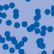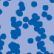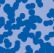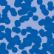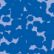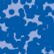## News

Prof. Dr. Karl-Theodor Sturm has been elected into the Academia Europaea. (28.06.2022)

Florian Schweiger erhielt den Hausdorff-Gedächtnispreis 2021 der Fachgruppe Mathematik für die beste Dissertation. Er fertigte die Dissertation unter der Betreuung von Prof. Stefan Müller an. Unter anderen wurde Vanessa Ryborz mit einem Preis der Bonner Mathematischen Gesellschaft für ihre von Prof. Sergio Conti betreute Bachelorarbeit ausgezeichnet. (18.01.2022)

Prof. Dr. Sergio Albeverio has been elected into the Academia Europaea and the Accademia Nazionale dei Lincei (more; 02.12.2021).

Der SFB 1060 Die Mathematik der emergenten Effekte hat eine dritte Förderperiode erhalten. (26.11.20)

Prof. Dr. Andreas Eberle erhält den diesjährigen Lehrpreis der Universität Bonn. (22.07.2020)

Herr Dr. Richard Höfer erhielt den Hausdorff-Gedächtnispreis 2019 der Fachgruppe Mathematik für die beste Disseration. Betreut wurde die Arbeit von Prof. J. Velázquez (29.01.2020).

Contact

Managing Director: Prof. Dr. Juan J. L. Velázquez